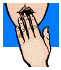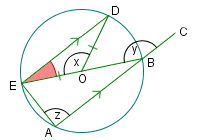Go to contentOne of the answers is wrong in the display below. Can you find it? And can you give the correct answer?You can get a better display of the maths by downloading special TeX fonts from jsMath. In the meantime, we will do the best we can with the fonts you have, but it may not be pretty and some equations may not be rendered correctly.

## Glossary

### union

The union of two sets A and B is the set containing all the elements of A and B.

Full Glossary List

## This question appears in the following syllabi:

SyllabusModuleSectionTopicExam Year
AQA AS Maths 2017Pure MathsStraight Lines and CirclesCircle Theorems-
AQA AS/A2 Maths 2017Pure MathsStraight Lines and CirclesCircle Theorems-
AQA GCSE (9-1) Higher (UK)G: Properties and ConstructionsG10: Circle TheoremsSpot the Mistake - Circle Theorems-
CBSE X (India)GeometryCirclesTangent perpendicular to radius-
CIE IGCSE (9-1) Maths (0626 UK)3 GeometryB3.7 Calculating Unknown AnglesSpot the Mistake - Circle Theorems-
Edexcel AS Maths 2017Pure MathsCirclesCircle Theorems-
Edexcel AS/A2 Maths 2017Pure MathsCirclesCircle Theorems-
Edexcel GCSE (9-1) Higher (UK)G: Properties and ConstructionsG10: Circle TheoremsSpot the Mistake - Circle Theorems-
GCSE Higher (UK)GeometryCirclesSpot the mistake-
OCR AS Maths 2017Pure MathsLines and CirclesCircle Theorems-
OCR GCSE (9-1) Higher (UK)8: Basic Geometry8.05: Circle TheoremsSpot the Mistake - Circle Theorems-
OCR MEI AS Maths 2017Pure MathsLines and CirclesCircle Theorems-
Universal (all site questions)CCirclesSpot the mistake-